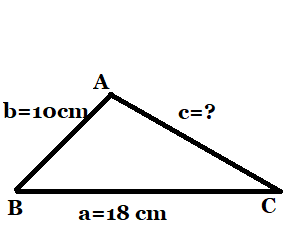October 1, 2023

# Find the area of a triangle two sides of which are 18cm and 10cm and the perimeter is 42cm

Class 9  Exercise 12.1  Question 4

find the area of a triangle two sides of which are 18cm and 10cm and the perimeter is  42cm .

Solution:  Let and

be the sides of the triangle  ABC.Here we have perimeter of the triangle    , and .

First we will find the 3rd side of the triangle  by using perimeter,  then we will use  Heron’s formula  to find the area of the  given triangle.

The Heron’s formula  for finding the area of a triangle  given by

 Area of a triangle =

where , and are the sides of the triangle and s= semi-perimeter i.e. half of the perimeter of the triangle= .

It is given that  . Putting the value of and , we get

18+10+=42

Now semi-perimeter

So

Therefore , area of the triangle =

=

=

Area of triangle =        .

Conclusion: The area of the triangle  two sides of which are 18 cm and 10 cm and the perimeter is
42 cm  is      .#### Bina singh

View all posts by Bina singh →
ssc chsl 2023 tier 1 cut off NIRF Rankings 2023 : Top 10 Engineering colleges in India CBSE Compartment Exam 2023 Application Form SSC CGL 2023 Notification: Important Dates UPSC Recruitment 2023